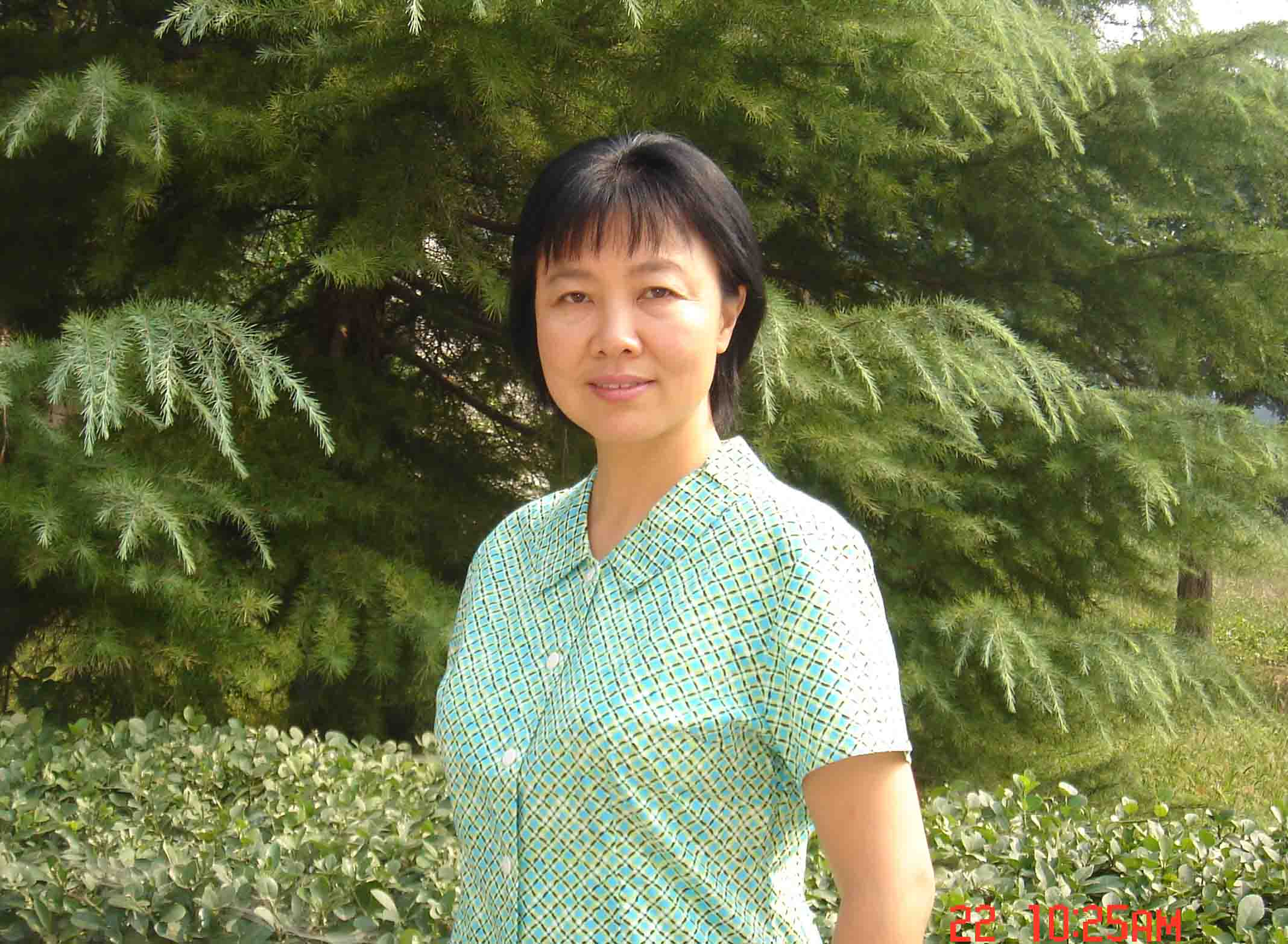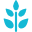#### 个人简介2000/10-至今，中国科学院，数学与系统科学研究院，研究员

1995/11-2000/09，中国科学院研究生院，博士生导师

1994/11-2000/09，中国科学院研究生院，教授

1991/09-1994/10，中国科学院研究生院，副教授

1989/05-1991/07，意大利理论物理国际中心，博士后

1987/10-1989/04，中国科学院研究生院，博士后

#### 研究方向#### 与几何有关的偏微分方程#### 与随机有关的偏微分方程

Fokker-Planck方程#### 学术论文

1. Ji, Min. An a priori estimate for Douglas problem in Riemannian manifolds. Acta Math. Sinica (N.S.) 5 (1989), no. 3, 235–249.

This paper is concerned with the Douglas problem in general Riemannian manifolds. For its perturbed problem we establish a uniform a priori estimate under the energy level min {d*,m* +s_{0}} (see Theorem 3.3). This level seems to be the best possible since in case of ℝn it is just the Douglas condition.

2. Ji, Min. A remark on the symmetry of solutions to nonlinear elliptic equations. Pacific J. Math. 153 (1992), no. 1, 157–162.

This note gives a necessary and sufficient condition for solutions of second order elliptic equations to be radially symmetric.

3. Ji, Min; Wang, Guang Yin. Minimal surfaces in Riemannian manifolds. Mem. Amer. Math. Soc. 104 (1993), no. 495, vi+50 pp.

A mulitiple solution theory to the Plateau problem in a Riemannian manifold is established. In S^n, the existence of two solutions to this problem is obtained. The Morse-Tompkins-Shiffman Theorem is extended to the case when the ambient space admits no minimal sphere.

4. Ji, Min. Minimal annuli in Riemannian manifolds. Acta Math. Sinica (N.S.) 9 (1993), no. 1, 74–89.

In this paper, a multiple solution theorem for minimal annuli coboundaries in a Riemannian manifold N is established. Especially, when the target manifold N is the standard sphere S^n, it implies the existence of at least two minimal annuli with given pair of wires (Γ1, Γ2) as their common boundaries θ.

5. Ji, Min. Multiple solutions to the Douglas problem in S^n. Sci. China Ser. A 36 (1993), no. 10, 1162–1168.

In S^3 a smooth Jordan curve Γ and a great circle σ which are mutually linked are shown to bound at least two minimal annuli. And in S^n(n≥3) it is proved that there exist at least two minimal annuli bounded by a smooth Jordan curve Γ and a great circle σ provided that σ is disjoint from Γ and intersects a least area surface spanning Γ.

6. Ji, Min. A simple construction of generalized harmonic maps discontinuous everywhere. Commun. Contemp. Math. 3 (2001), no. 3, 355–362.

This paper constructs an everywhere discontinuous harmonic map from a domain of dimension 2 into S^1. Our construction is simpler than that of L. Almeida.

7. Bao, Jiguang; Chen, Jingyi; Guan, Bo; Ji, Min. Liouville property and regularity of a Hessian quotient equation. Amer. J. Math. 125 (2003), no. 2, 301–316.

We are concerned with properties of (convex) solutions to the Hessian quotient equation Sn,k(D^2u) = φ, 1 ≤ k < n. As our first main result we prove some regularity of strong solutions, while the second states that for φ ≡ 1 an entire convex solution with a quadratic growth bound must be a quadratic polynomial.

8. Ji, Min. On positive scalar curvature on S^2. Calc. Var. Partial Differential Equations 19 (2004), no. 2, 165–182.

We consider the problem of prescribing scalar curvature on the standard 2-sphere S^2. It is proved that any positive smooth function on S^2 is the scalar curvature of some pointwise conformal metric, if an associated map has non-zero degree. As a result we improve some previous important results and give some completely new ones.

9. Ji, Min. Scalar curvature equation on S^n. I. Topological conditions. J. Differential Equations 246 (2009), no. 2, 749–787.

This is the first part of a series devoting to the study of the prescribing scalar curvature problem on the standard sphere of any dimension. In the first part, we will adopt the degree-theoretic approach to give a topological condition and some general, explicit conditions on the scalar curvature functions to ensure the solvability of the problem. Our topological condition is imposed on some of simple maps explicitly defined by the scalar curvature function, which is derived from the asymptotic expansion of the boundary map introduced in [A. Chang, P. Yang, A perturbation result in prescribing scalar curvature on S^n, Duke Math. J. 64 (1991) 27–69]. Our conditions, particularly allowing non-isolation and non-degeneracy of the critical points of the scalar curvature functions, can be easily verified in many situations. In the second part of series, we will make a detailed study on the verification of the topological condition. Our results will generalize almost all existing ones in the same direction and meanwhile provide a unified treatment for both symmetric and non-symmetric cases of the scalar curvature functions.

10. Ji, Min. Scalar curvature equation on S^n. II. Analytic characterizations. J. Differential Equations 246 (2009), no. 2, 788–818.

This is the second part of a series devoting to the study of the prescribing scalar curvature problem on the standard sphere of any dimension. By studying topological degrees for certain abstract maps, we will give explicit analytic conditions on the scalar curvature function which verify the topological degree conditions given in the first part of the series to ensure the solvability of the problem. General existence results for the prescribing scalar curvature equation will be given on both H-symmetric and sub-H-symmetric solutions corresponding to H-symmetric scalar curvature functions, as well as on non-symmetric solutions corresponding to symmetric-like scalar curvature functions. Special axisymmetric and axisymmetric-like cases will be also considered. Our analysis will be based on a general approach of dimension reductions and degree calculations by taking advantage of symmetries and symmetric-like properties.

11. Huang, Wen; Ji, Min; Liu, Zhenxin; Yi, Yingfei. Integral identity and measure estimates for stationary Fokker-Planck equations. Ann. Probab. 43 (2015), no. 4, 1712–1730.

We consider a Fokker–Planck equation in a general domain in R^n with L^{p)_{loc} drift term and W^{1,p}_{loc} diffusion term for any p>n. By deriving an integral identity, we give several measure estimates of regular stationary measures in an exterior domain with respect to diffusion and Lyapunov-like or anti-Lyapunov-like functions. These estimates will be useful to problems such as the existence and nonexistence of stationary measures in a general domain as well as the concentration and limit behaviors of stationary measures as diffusion vanishes.

12. Huang, Wen; Ji, Min; Liu, Zhenxin; Yi, Yingfei. Steady states of Fokker-Planck equations: I. Existence. J. Dynam. Differential Equations 27 (2015), no. 3-4, 721–742.

This is the first paper in a series concerning the study of steady states of a Fokker–Planck equation in a general domain in R^n with L^{p}_{loc} drift term and W^{1,p}_{loc} diffusion term for any p>n . In this paper, by using the level set method especially the integral identity which we introduced in Huang et al. (Ann Probab, 2015), we obtain several new existence results of steady states, including stationary solutions and measures, of the Fokker–Planck equation with non-degenerate diffusion under Lyapunov-like conditions. As applications of these results, we give some examples on the noise stabilization of an unstable equilibrium and the existence and uniqueness of steady states subject to boundary degeneracy of diffusion in a bounded domain.

13. Huang, Wen; Ji, Min; Liu, Zhenxin; Yi, Yingfei. Steady states of Fokker-Planck equations: II. Non-existence. J. Dynam. Differential Equations 27 (2015), no. 3-4, 743–762.

This is the second paper in a series concerning the study of steady states, including stationary solutions and measures, of a Fokker–Planck equation in a general domain in R^n with L^{p}_{loc} drift term and W^{1,p}_{loc} diffusion term for any p>n . In this paper, we obtain some non-existence results of stationary measures under conditions involving anti-Lyapunov type of functions associated with the stationary Fokker–Planck equation. When combined with the existence results showed in part I of the series (Huang et al. in J. Dyn Differ Equ 10.1007/s10884-015-9454-x, 2015) contained in the same volume, not only will these results yield necessary and sufficient conditions for the existence of stationary measures, but also they provide a useful tool for one to study noise perturbations of systems of ordinary differential equations, especially with respect to problems of stochastic bifurcations, as demonstrated in some examples contained in this paper. Our analysis is based on the level set method, in particular the integral identity, and measure estimates contained in our work (Huang et al. in Ann Probab 43:1712–1730, 2015).

14. Ji, Min. Projective Dirichlet boundary condition with applications to a geometric problem. Acta Math. Sin. (Engl. Ser.) 32 (2016), no. 1, 11–24.

Given a domain Ω ⊂ R^n, let λ > 0 be an eigenvalue of the elliptic operator L:= ∑^{n}_{i,j=1}(∂/∂x_{i})(a^{ij}∂/∂x_{j}) on Ω for Dirichlet condition. For a function f ∈ L^2(Ω), it is known that the linear resonance equation Lu + λu = f in Ω with Dirichlet boundary condition is not always solvable. We give a new boundary condition P_{λ}(u|∂Ω) = g, called to be projective Dirichlet condition, such that the linear resonance equation always admits a unique solution u being orthogonal to all of the eigenfunctions corresponding to λ which satisfies ‖u‖_{2,2} ≤ C(‖f‖_{2} + ‖g‖_{2,2}) under suitable regularity assumptions on ∂Ω and L, where C is a constant depends only on n, Ω, and L. More a priori estimates, such as W^{2,p}-estimates and the C^{2,α}-estimates etc., are given also. This boundary condition can be viewed as a generalization of the Dirichlet condition to resonance equations and shows its advantage when applying to nonlinear resonance equations. In particular, this enables us to find the new indicatrices with vanishing mean (Cartan) torsion in Minkowski geometry. It is known that the geometry of indicatries is the foundation of Finsler geometry.

15. Huang, Wen; Ji, Min; Liu, Zhenxin; Yi, Yingfei. Steady states of Fokker-Planck equations: III. Degenerate diffusion. J. Dynam. Differential Equations 28 (2016), no. 1, 127–141

This is the third paper in a series concerning the study of steady states of a Fokker–Planck equation in a general domain in R^n with L^{p}_{loc} drift term and W^{1,p}_{loc} diffusion term for any p>n. In this paper, we give some existence results of stationary measures of the Fokker–Planck equation under Lyapunov conditions which allow the degeneracy of diffusion.